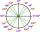# Regular n-gon

In a regular n-angle polygon the internal angle is 144 degrees. Find the number n indicating the number of sides of this polygon.

Result

n =  10

#### Solution:Leave us a comment of example and its solution (i.e. if it is still somewhat unclear...):

Showing 0 comments:Be the first to comment!## Next similar examples:

1. Reference angleFind the reference angle of each angle:
2. SlopeFind the slope of the line: x=t and y=1+t.
3. Radians compareWhat is greater angle? -0.7 radians or -0.7π radians?
4. Expression with powersIf x-1/x=5, find the value of x4+1/x4
5. Nineteenth memberFind the nineteenth member of the arithmetic sequence: a1=33 d=5 find a19
6. 6 termsFind the first six terms of the sequence. a1 = 7, an = an-1 + 6
7. Theorem proveWe want to prove the sentense: If the natural number n is divisible by six, then n is divisible by three. From what assumption we started?
8. Volleyball8 girls wants to play volleyball against boys. On the field at one time can be six players per team. How many initial teams of this girls may trainer to choose?
9. Holidays - on poolChildren's tickets to the swimming pool stands x € for an adult is € 2 more expensive. There was m children in the swimming pool and adults three times less. How many euros make treasurer for pool entry?
10. MeanA student food the mean of 50 items as 38.6. When checking the work he found that he had taken one item as 50 why it should correctly read as 40 in this circumstances. What should be the correct mean?
11. PowersExpress the expression ? as the n-th power of the base 10.
12. Factory and divisionsThe factory consists of three auxiliary divisions total 2,406 employees. The second division has 76 employees less than 1st division and 3rd division has 212 employees more than the 2nd. How many employees has each division?
13. One halfOne half of ? is: ?
14. TreesA certain species of tree grows an average of 0.5 cm per week. Write an equation for the sequence that represents the weekly height of this tree in centimeters if the measurements begin when the tree is 200 centimeters tall.
15. Linear systemSolve a set of two equations of two unknowns: 1.5x+1.2y=0.6 0.8x-0.2y=2
16. Two equationsSolve equations (use adding and subtracting of linear equations): -4x+11y=5 6x-11y=-5
17. CoefficientDetermine the coefficient of this sequence: 7.2; 2.4; 0.8